# Samacheer Kalvi 6th Maths Solutions Term 3 Chapter 3 Perimeter and Area Ex 3.2## Tamilnadu Samacheer Kalvi 6th Maths Solutions Term 3 Chapter 3 Perimeter and Area Ex 3.2

Miscellaneous Practice Problems

Question 1.
A piece of wire is 36 cm long. What will be the length of each side if we form
i) a square
ii) an equilateral triangle.
Solution:
Given the length of the wire = 36 cm
i) When a square is formed out of it
The perimeter of the square = 36 cm
4 × side = 36
side = $$\frac{36}{4}$$ = 9 cm
Side of the square

ii) When an equilateral triangle is formed out of it, its perimeter = 36 cm
i.e., side + side + side = 36 cm .
3 × side = 36 cm
side = $$\frac{36}{3}$$ = 12 cm
One side of an equilateral triangle = 12 cm

Question 2.
From one vertex of an equilateral triangle with side 40 cm, an equilateral triangle with 6 cm side is removed. What is the perimeter of the remaining portion?
Solution: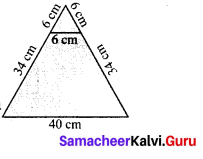If an equilateral triangle of side 6 cm is removed the perimeter = (40 + 34 + 6 +34) cm = 114 cm
Perimeter of the remaining portion = 114 cm

Question 3.
Rahim and peter go for a morning walk, Rahim walks around a square path of side 50 m and Peter walks around a rectangular path with length 40 m and breadth 30 m. If both of them walk 2 rounds each, who covers more distance and by how much?
Solution:
Distance covered by Rahim
= 50 × 4 m
= 200 m
If he walks 2 rounds, distance covered = 2 × 200 m
= 400 m
Distance covered by peter
= 2 (40 + 30) m
= 2(70)m
= 140 m
If he walks 2 rounds, distance covered = 2 × 140 m
= 280 m
∴ Rahim covers more distance by (400 – 280) = 120 mQuestion 4.
The length of a rectangular park is 14 m more than its breadth. If the perimeter of the park is 200 m, what is its length? Find the area of the park?
Solution:
Given length of rectangular park is 14m more than its breadth.
Let the breadth be b m .
∴ Length of the park will be l = b + 14 m
Given perimeter = 200 m
2 × (l + b) = 200 m
2 × (b + 14 + b) = 200 m [∵ l = b + 14]
2 × (2b + 14) = 200 m
2b + 14 = $$\frac{200}{2}$$ m
2b + 14 = 100 m
2b = 100 – 14 m
2b = 86 m
b = $$\frac{86}{2}$$ m
b 43 m
Length Length of the park = 57 m
Area of a rectangle = (length × breadth) unit2
= (57 × 43) m2 = 2,451 m2
Area of the park = 2,451 m2

Question 5.
Your garden is in the shape of a square of side 5 m. Each side is to be fenced with 2 rows of wire. Find how much amount is needed to fence the garden at Rs 10 per meter.
Solution:
a = 5 m
Perimeter of the garden
= 4 a units
= 4 × 5 m
= 20 m
For 1 row
Amount needed to fence l m= Rs 10
Amount needed to fence 20 m
= Rs 10 × 20
= Rs 200
For 2 rows
Total amount needed = 2 × Rs 200
= Rs 400

Challenge Problems

Question 6.
A closed shape has 20 equal sides and one of its sides is 3 cm. Find its perimeter.
Solution:
Number of equal sides in the shape = 20
One of its side = 3 cm
Perimeter = length of one side × Number of equal sides
∴ Perimeter = (3 × 20) cm = 60 cm
∴ Perimeter = 60 cm

Question 7.
A rectangle has length 40 cm and breadth 20 cm. How many squares with side 10 cm can be formed from it.
Solution:
l = 40 cm, b = 20 cm
Area of the rectangle = l × b sq units
= 40 × 20 cm²
= 800 cm²
a = 10 cm
Area of the square = a × a sq. units
= 10 × 10 cm²
= 100 m²
No of squares formed = $$\frac{800}{100}$$ cm²
= 8

Question 8.
The length of a rectangle is three times its breadth. If its perimeter is 64 cm, find the sides of the rectangle.
Solution:
Given perimeter of a rectangle = 64 cm
Also given length is three times its breadth.
Let the breadth of the rectangle = b cm
∴ Length = 3 × b cm
Perimeter = 64 m
i.e., 2 × (l + b) = 64 m
2 × (3b + b) = 64 m
2 × 4b = 64m
4b = $$\frac{64}{2}$$ = 32 m
b = $$\frac{32}{4}$$ = 8 m
l = 3 × b = 3 × 8 = 24 m
∴ Breadth of the rectangle = 8 m
Length of the rectangle = 24 m

Question 9.
How many different rectangles can be made with a 48 cm long string? Find the possible pairs of length and breadth of the rectangles.
Solution:
12 rectangles
(1, 23), (2, 22), (3, 21), (4, 20), (5, 19), (6, 18), (7, 17), (8, 16), (9, 15), (10, 14), (11, 13), (12, 12)Question 10.
Draw a square B whose side is twice of the square A. Calculate the perimeters of the squares A and B.
Solution: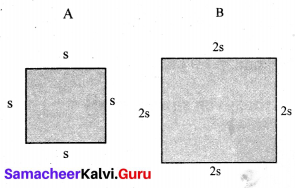Perimeter of A = s + s + s + s units = 4 s units
Perimeter of B = (2s + 2s + 2s + 2s) units
= 8s units = 2 (4s) units.
∴ Perimeter of B is twice perimeter of A

Question 11.
What will be the area of a new square formed if the side of a square is made one – fourth?
Solution:
Area of the new square is reduced to $$\frac{1}{16}$$ th times to that of the original area.

Question 12.
Two plots have the same perimeter. One is a square of side 10 m and another is a rectangle of breadth 8 m. Which plot has the greater area and by how much?
Solution: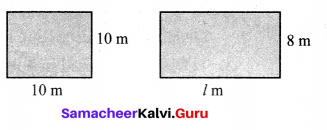Given perimeter of square = perimeter of rectangle
4 × side = 2 (length + breadth)
(4 × 10) m = 2(l + 8)m
$$\frac{4 \times 10}{2}$$ = l + 8
20 = l + 8
l = 20 – 8
l = 12 m
∴ length of the rectangle = 12 m
Area of the square plot – side × side = 10 × 10 m2 = 100 m2
Area of the rectangular plot = length × breadth = (12 × 8) m2 = 96 m2
100 m2 > 96 m2
∴ Square plot has greater area by 4m2

Question 13.
Look at the picture of the house given and find the total area of the shaded portion.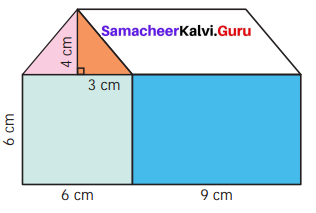Solution:
Total area of the shaded region = Area of a right triangle + Area of a rectangle
= ($$\frac{1}{2}$$ × b × h) + (l × b) cm2
= [($$\frac{1}{2}$$ × 3 × 4) + (9 × 6)] cm2
= (6 + 54) cm2 = 60 cm2

Question 14.
Find the approximate area of the flower in the given square grid.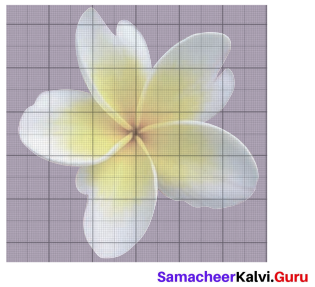Solution:
Approximate area = Number of full squares + Number of more than half squares + $$\frac{1}{2}$$ × Number of half squares.
= 10 + 5 + ($$\frac{1}{2}$$ × 1) Sq units. = 10 + 5 + $$\frac{1}{2}$$ sq. units
= 15 $$\frac{1}{2}$$ sq. units = 15.5 sq. units.